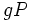Sylow implies order-dominating

This article gives the statement and possibly, proof, of an implication relation between two subgroup properties, when the big group is a finite group. That is, it states that in a Finite group (?), every subgroup satisfying the first subgroup property (i.e., Sylow subgroup (?)) must also satisfy the second subgroup property (i.e., Order-dominating subgroup (?)). In other words, every Sylow subgroup of finite group is a order-dominating subgroup of finite group.
View all subgroup property implications in finite groups$|$ View all subgroup property non-implications in finite groups$|$ View all subgroup property implications$|$ View all subgroup property non-implications

Statement

Property-theoretic statement

The subgroup property of being a Sylow subgroup is stronger than, or implies, the subgroup property of being an order-dominating subgroup: for any subgroup whose order divides the order of the Sylow subgroup, some conjugate of that subgroup is contained in the given Sylow subgroup.

Statement with symbols

Suppose$G$ is a finite group and$p$ is a prime number. Then, if$P$ is a$p$-Sylow subgroup and$Q$ is a$p$-subgroup of$G$, there exists$g \in G$ such that$g^{-1}Qg \le P$.

This statement is a part of Sylow's theorem.

Proof

Proof using coset spaces

Given:$G$ a finite group,$P$ a$p$-Sylow subgroup, and$Q$ a$p$-group. Suppose$n=p^rm$ where$n$ is the order of$G$ and$m$ is relatively prime to$p$ (So,$|P| = p^r$.

To prove: There exists$g \in G$ such that$g^{-1}Qg \le P$.

Proof: We prove this through a series of observations:

•$G$ naturally acts (on the left) on the left coset space of$P$.
• Since$Q$ is a subgroup of$G$,$Q$ also acts on the left coset space of$P$
• The left coset space of$P$ has cardinality$m$, which is relatively prime to$p$. Hence, under the action of$Q$ (which is a$p$-group) on this set, there is at least one fixed point. Let the fixed point be$gP$.
• We have$QgP = gP$. This gives us:$(g^{-1}Qg)P = P$, and hence$g^{-1}Qg \subseteq P$. Thus, a conjugate of$Q$ is contained inside$P$.